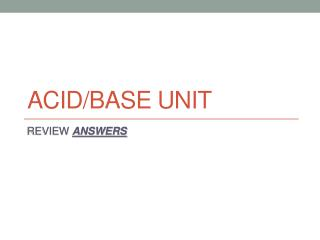DownloadDownload PresentationAcid/Base Unit

# Acid/Base Unit

Télécharger la présentation## Acid/Base Unit

- - - - - - - - - - - - - - - - - - - - - - - - - - - E N D - - - - - - - - - - - - - - - - - - - - - - - - - - -
##### Presentation Transcript

2. Practice defining acids and bases. WORTH YOUR TIME? 16 pts on 100 pt. test Looking at the formula Al(OH)3, we would assume that • this is a base • this has a pH of 7 • this is an acid • the concentration is stable According to the Bronsted-Lowry model, an base is a(n) • H+ acceptor • proton donor • H+ ion producer • OH- ion producer • none of these Which represents a conjugate acid-base pair? • H2O, H3O+ • OH-, HNO3 • H2SO4, SO42- • HC2H3O2, C2H3O2- Identify the acid, base, conjugate acid, and conjugate base. H2SO4 + H2O --> H3O+ + HSO4- A B CA CB NH3 + H2O --> OH- + NH4+ B A CB CA

3. Differentiate between strong acid and weak acid. • Individually: • Read the following statement: Acids dissociate in water to form H+ ions. Some acids dissociate more readily than others. Strong acids dissociate completely to form the most H+ ions possible; while weak acids only dissociate partially. • With your Partner: • Where did you hear “strong acid” or “weak acid” used in this unit? • Choose the correct answer: Solid hydrogen sulfate partially dissociates when added to water to produce an aqueous solution of HSO4- and H3O+. Therefore, sulfuric acid is a • strong acid • weak acid • conjugate acid • none of these

4. Understanding the Kw equation • Individually: • Write the kw equation. 1.0x10-14 = [H+][OH-] • Write a sentence that explains when this equation should be used. • With your Partner: • Check your equation. • Compare your explanations of the usefulness of this equation. • Worth your time? • 14 pts to 30 pts on 100 pt test

5. Understanding the pH equation • Individually: • Write the pH equation. pH = -log[H+] • Write a sentence that explains when this equation should be used. • Fill in the blanks: The pH scale goes from 0 to 14. Acids have a pH below7, and bases have a pH above7. • With your Partner: • Check your equation. • Compare your explanations and answers to the fill in the blank statement. • Worth your time? • 21 pts on 100 pt test

6. Understanding the pOH equation • Individually: • Write the pOH equation. pOH = -log[OH-] • Write a sentence that explains when this equation should be used. • How is the pOH equation different from the pH equation? • With your Partner: • Check your equation. • Compare your explanations. • Worth your time? • 16 pts on 100 pt test

7. Understanding the relationship between pH and pOH • Individually: pH + pOH = 14 • Write the equation that includes both pH and pOH. • Write a sentence that explains when this equation should be used. • With your Partner: • Check your equation. • Compare your explanations. • Worth your time? • 16 pts on 100 pt test

8. Practice Kw, pH, and pOH calculations. • Check your answers to yesterday’s handout. • Solve. • During the course of the day, human saliva varies between acidic and basic. What is the [H+] in saliva if [OH-] is 3.16x10-8M? What is the pH of the saliva? At this point in the day, is it acidic, basic, or neutral? [H+] =3.16x10-7 M, pH = 6.5, acidic • What is the concentration of OH- ions in 0.1M bicarbonate of soda if [H3O+] is 3.98x10-9M? Is the bicarbonate acidic, basic, or neutral? What is the pH? What is the pOH? [OH-]=2.51x10-6M, pOH=5.6, pH=8.4, basic • What is the concentration of H3O+ ions in eggs if [OH-] is 6.0x10-7M? Are eggs acidic, basic, or neutral? [H+] = 1.67x10-8 M, basic • If the pH of a solution is 6.4, what is the [OH-]? [OH-] = 2.51x10-8 M • Find the [H+] and [OH-] of a solution with a pH of 3.494. [H+] = 3.2x10-4 M, [OH-] =3.1x10-11 M • For each pair of concentrations, tell which represents the more acidic solution. • [H+] = 0.000013 M or [OH-] = 0.0000032 M • [H+] = 1.03x10-6 M or [OH-] = 1.54x10-8 M

9. Understanding titrations • Individually: • Fill in the blanks. Every time that an acid and a base react in a neutralization reaction, a saltand waterare produced. • Solve: A volume of 20-mL of 0.25M Al(OH)3 neutralizes a 75-mL sample of H2SO4 solution. What is the concentration of the acid? • 2Al(OH)3 + 3H2SO4 6H2O + Al2(SO4)3, Acid M = 0.1 M • Solve: Calculate how many milliliters of 0.30M H2SO4 must be added to titrate 75 mL of 0.10 M KOH. • H2SO4 + 2KOH  2H2O + K2SO4, 0.025 L or 25mL of acid • With your Partner: • Check your fill in the blank statement. • Compare your balanced equations with your partner. • Compare your work and answers to the two calculations. • NOTE: IF SOMEONE HAS TOLD YOU ABOUT A SHORT-CUT (MAVA = MBVB), FORGET IT. IT DOES NOT WORK CONSISTENTLY. • Worth your time? • 10 pts on 100 pt test